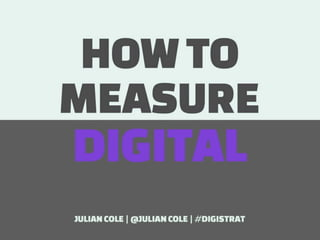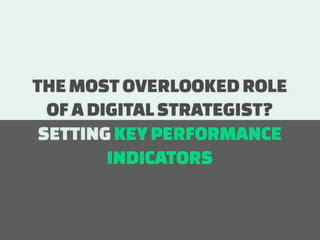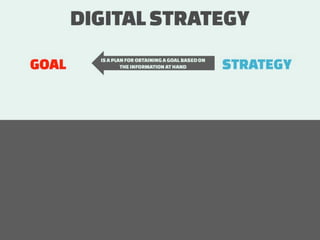Successfully reported this slideshow.

# How to measure Digital

292

Share×
1 of 71
1 of 71

# How to measure Digital

292

Share

#### Description

How to measure Digital looks at the important role of Key Performance Indicators and how you create them. It takes a Communication Objective and turns it into Strategy that leads to Tactics to support the Strategy. The final piece is putting in place Key Performance Indicators which makes up the parts of the Communications Objective.

• \n
• \n
• \n
• \n
• \n
• \n
• \n
• \n
• \n
• \n
• \n
• \n
• \n
• \n
• \n
• \n
• \n
• \n
• \n
• \n
• \n
• \n
• \n
• \n
• \n
• \n
• \n
• \n
• \n
• \n
• \n
• \n
• \n
• \n
• \n
• \n
• \n
• \n
• \n
• \n
• \n
• \n
• \n
• \n
• \n
• \n
• \n
• \n
• \n
• \n
• \n
• \n
• \n
• \n
• \n
• \n
• \n
• \n
• \n
• \n
• \n
• \n
• \n
• \n
• \n
• \n
• \n
• \n
• \n
• \n
• \n
• #### Description

How to measure Digital looks at the important role of Key Performance Indicators and how you create them. It takes a Communication Objective and turns it into Strategy that leads to Tactics to support the Strategy. The final piece is putting in place Key Performance Indicators which makes up the parts of the Communications Objective.

• \n
• \n
• \n
• \n
• \n
• \n
• \n
• \n
• \n
• \n
• \n
• \n
• \n
• \n
• \n
• \n
• \n
• \n
• \n
• \n
• \n
• \n
• \n
• \n
• \n
• \n
• \n
• \n
• \n
• \n
• \n
• \n
• \n
• \n
• \n
• \n
• \n
• \n
• \n
• \n
• \n
• \n
• \n
• \n
• \n
• \n
• \n
• \n
• \n
• \n
• \n
• \n
• \n
• \n
• \n
• \n
• \n
• \n
• \n
• \n
• \n
• \n
• \n
• \n
• \n
• \n
• \n
• \n
• \n
• \n
• \n
• ## More Related Content

### Related Books

Free with a 30 day trial from Scribd

See all

### Related Audiobooks

Free with a 30 day trial from Scribd

See all